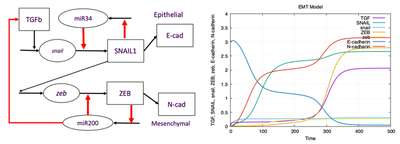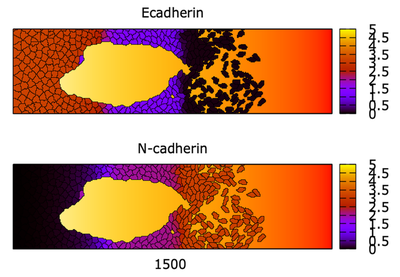# MDCK EMT D

##### Persistent Identifier

Multiscale model of epithelial-mesenchymal transition (EMT) with intracellular TGFβ signaling circuit

## Introduction

The growth factor TGFβ regulates the snail-zeb intracellular circuit that affects EMT by controlling the levels of E and N-cadherin expression. One popular model for this circuit, due to Tian et al. (2013) is shown on this page. TGFβ upregulates SNAIL1 that promotes ZEB, while depressing itself through miRNA34. SNAIL1 and ZEB respectively promote the expression of E-cadherin and N-cadherin.

## Description

We first constructed an exact replica of this full Tian et al. (2013) ODE model in Morpheus, then ran simulations for various levels of exogenous TGFβ, the growth factor that stimulates the EMT process. As shown in the following figure, results are concordant with Figure 3A of Tian et al. (2013). A normal epithelial state exists, in the absence of exogenous TGFβ. This state consists of high E-cad and low levels of all other effectors. Increasing the exogenous TGFβ to $1.5$ results in an EM state that has moderate levels of both E- and N-cadherins. A further increase to $3$ leads to the ‘fully mesenchymal’ state where N-cadherin dominates and E-cadherin has been reduced to very low levels.A TGFβ EMT signaling circuit: A schematic diagram of the circuit as implemented by Tian et al. (2013) (left). Setting $\mathrm{TGF}_0 = 3$, leads to a mesenchymal state with very low E-cad and high N-cad (right). Produced by Morpheus file TianODEs_main.xml with equations and parameter values kindly supplied by Xiao-Jun Tian.

In the second stage, the TGFβ intracellular circuit was inserted in our wound-healing cell sheet simulation in place of the YAP circuit. The following minimal modifications were made to adjust the multiscale model to the new circuit:

1. High N-cad cells were assumed to be ‘more mesenchymal’.
2. Cell-cell adhesion was assumed to depend only on the level of E-cadherin, as before.
3. A static gradient of exogenous TGFβ was simulated. The value of $\mathrm{TGF}_ 0 = \mathrm{TGFβ} (x, y)$ was assumed to directly feed into the level of $\mathrm{TGF}_ 0$ in any cell at the given position $(x, y)$ in the 2D domain. Mesenchymal cells were assumed to move faster and have longer shapes. To Implement this, we assumed that DirectedMotion[strength]$\ = 1 + \frac{1}{3} N_\mathrm{cad}\vec{u}$, where $\vec{u}$ is an unit vector in the $x$ direction. A LengthConstraint is also implemented in the Hamiltonian, changing the cells to slightly longer oval shapes rather than polygonal morphology at low N-cadherin.β cell sheet morphology: Epithelial, mesenchymal, and intermediate states in the TGFβ model by Tian et al. (2013). Shown is a static exogenous TGF gradient (orange background, $\mathrm{TGF}_0$ in model) and cell sheet morphology for parameter values that produced the ODE simulations. Top: E-cadherin level. Bottom: N-cadherin level. As cells migrate into the high TGFβ level, their E-cadherin decreases, so cells break away and become a swarm of mesenchymal cells. Where TGFβ is low (left end of domain), E-cadherin is high enough that all cells are epithelial. An intermediate EM state is seen at moderate levels of TGFβ, in the model of the domain. Morpheus file: TianTGFbetaCellSheet.xml.

## Results

At high TGFβ, N-cadherin dominates, and the cells are all mesenchymal. A transition zone with partial EM state is also seen in the domain, where both E- and N-cadherins are at moderate levels. Collective, rather than single cell migration is seen in that zone.

Video of the model simulation TianTGFbetaCellSheet.xml: Animated results from the multiscale model derived from the TGFβ EMT signaling circuit.

## Reference

This model is the original used in the publication, up to technical updates:

N. Mukhtar, E. N. Cytrynbaum, L. Edelstein-Keshet: A multiscale computational model of YAP signaling in epithelial fingering behavior. Biophys. J. 121 (10): 1940-1948, 2022.

## Model

Get this model via:

•  Download: TianODEs_main.xml
• XML Preview

<?xml version='1.0' encoding='UTF-8'?>
<MorpheusModel version="4">
<Description>
<Details>Naba Mukhtar, Eric N Cytrynbaum, and Leah Edelstein-Keshet (2022) A Multiscale computational model of YAP signaling in epithelial fingering behaviour

Produced SI Figure  13

ODE model for the Tian et al model for epithelial-mesenchymal transition (EMT)
governed by TGF-beta.

Tian, X.J., Zhang, H. and Xing, J. (2013)
Coupled reversible and irreversible bistable switches
underlying TGFβ-induced epithelial to mesenchymal transition.
Biophysical journal, 105(4), pp.1079-1089.

This file was constructed by Leah Edelstein-Keshet using
an XPP .ode file kindly supplied by Prof. Xiaojun Tian.
</Details>
<Title>TianODEs</Title>
</Description>
<Time>
<StartTime value="0"/>
<StopTime value="500" symbol="stoptime"/>
<TimeSymbol symbol="time"/>
<RandomSeed value="1"/>
</Time>
<Space>
<Lattice class="linear">
<Size value="1, 0, 0" symbol="size"/>
<Neighborhood>
<Order>1</Order>
</Neighborhood>
</Lattice>
<SpaceSymbol symbol="space"/>
</Space>
<Global>
<Variable name="TGF" value="0" symbol="TGF"/>
<Variable name="snail" value="0.01" symbol="snail"/>
<Variable name="SNAIL" value="0.01" symbol="SNAIL"/>
<Variable name="miR34" value="0.4" symbol="miR34"/>
<Variable name="zeb" value="0.01" symbol="zeb"/>
<Variable name="ZEB" value="0.01" symbol="ZEB"/>
<Variable name="miR200" value="0.4" symbol="miR200"/>
<System solver="Runge-Kutta [fixed, O(4)]" time-step="0.1">
<Constant name="TGF0" value="3" symbol="TGF0"/>
<Constant name="k0TGF" value="0.06" symbol="k0TGF"/>
<Constant name="kTGF" value="1.2" symbol="kTGF"/>
<Constant name="JTGF" value="0.06" symbol="JTGF"/>
<Constant name="kdTGF" value="0.6" symbol="kdTGF"/>
<Constant name="Jsnail" value="1.6" symbol="Jsnail"/>
<Constant name="kdsnail" value="0.09" symbol="kdsnail"/>
<Constant name="k0snail" value="0.0006" symbol="k0snail"/>
<Constant name="ksnail" value="0.03" symbol="ksnail"/>
<Constant name="kSNAIL" value="17.0" symbol="kSNAIL"/>
<Constant name="JSNAIL" value="0.08" symbol="JSNAIL"/>
<Constant name="kdSNAIL" value="1.66" symbol="kdSNAIL"/>
<Constant name="k034" value="0.0012" symbol="k034"/>
<Constant name="k34" value="0.012" symbol="k34"/>
<Constant name="J134" value="0.15" symbol="J134"/>
<Constant name="J234" value="0.36" symbol="J234"/>
<Constant name="kd34" value="0.035" symbol="kd34"/>
<Constant name="k0zeb" value="0.003" symbol="k0zeb"/>
<Constant name="kzeb" value="0.06" symbol="kzeb"/>
<Constant name="Jzeb" value="3.5" symbol="Jzeb"/>
<Constant name="kdzeb" value="0.09" symbol="kdzeb"/>
<Constant name="kZEB" value="17" symbol="kZEB"/>
<Constant name="JZEB" value="0.06" symbol="JZEB"/>
<Constant name="kdZEB" value="1.66" symbol="kdZEB"/>
<Constant name="k02" value="0.0002" symbol="k02"/>
<Constant name="k2" value="0.012" symbol="k2"/>
<Constant name="kd2" value="0.035" symbol="kd2"/>
<Constant name="J12" value="5.0" symbol="J12"/>
<Constant name="J22" value="0.2" symbol="J22"/>
<DiffEqn symbol-ref="TGF" name="Equation for TGF">
<Expression>k0TGF+kTGF/(1+(miR200/JTGF)^2)-kdTGF*TGF</Expression>
</DiffEqn>
<DiffEqn symbol-ref="snail" name="Equation for snail">
<Expression>k0snail+ksnail*(((TGF+TGF0)/Jsnail)^2)/(1+((TGF+TGF0)/Jsnail)^2)-kdsnail*snail</Expression>
</DiffEqn>
<DiffEqn symbol-ref="SNAIL" name="Equation for SNAIL">
<Expression>kSNAIL*snail/(1+(miR34/JSNAIL)^2)-kdSNAIL*SNAIL</Expression>
</DiffEqn>
<DiffEqn symbol-ref="miR34" name="Equation for miR34">
<Expression>k034+k34/(1+(SNAIL/J134)^2+(ZEB/J234)^2)-kd34*miR34</Expression>
</DiffEqn>
<DiffEqn symbol-ref="zeb" name="Equation for zeb">
<Expression>k0zeb+kzeb*(SNAIL/Jzeb)^2/(1+(SNAIL/Jzeb)^2)-kdzeb*zeb</Expression>
</DiffEqn>
<DiffEqn symbol-ref="ZEB" name="Equation for ZEB">
<Expression>kZEB*zeb/(1+(miR200/JZEB)^2)-kdZEB*ZEB</Expression>
</DiffEqn>
<DiffEqn symbol-ref="miR200" name="Equation for miR200">
<Expression>k02+k2/(1+(SNAIL/J12)^2+(ZEB/J22)^2)-kd2*miR200</Expression>
</DiffEqn>
</DiffEqn>
</DiffEqn>
</System>
</Global>
<Analysis>
<Logger time-step="0.1">
<Plots>
<Plot title="EMT Model" time-step="-1">
<Style decorate="true" style="lines" line-width="2.0" grid="true"/>
<Terminal terminal="png"/>
<X-axis>
<Symbol symbol-ref="time"/>
</X-axis>
<Y-axis minimum="0.0" maximum="4">
<Symbol symbol-ref="TGF"/>
<!--    <Disabled>
<Symbol symbol-ref="miR200"/>
</Disabled>
-->
<!--    <Disabled>
<Symbol symbol-ref="miR34"/>
</Disabled>
-->
<Symbol symbol-ref="SNAIL"/>
<Symbol symbol-ref="snail"/>
<Symbol symbol-ref="ZEB"/>
<Symbol symbol-ref="zeb"/>
</Y-axis>
</Plot>
</Plots>
<Output>
<TextOutput/>
</Output>
<Input/>
</Logger>
<ModelGraph include-tags="#untagged" format="dot" reduced="false"/>
</Analysis>
</MorpheusModel>



• TianODEs_main.xml (produced SI Fig. S13) and
• TianTGFbetaCellSheet.xml (produced SI Fig. S14),